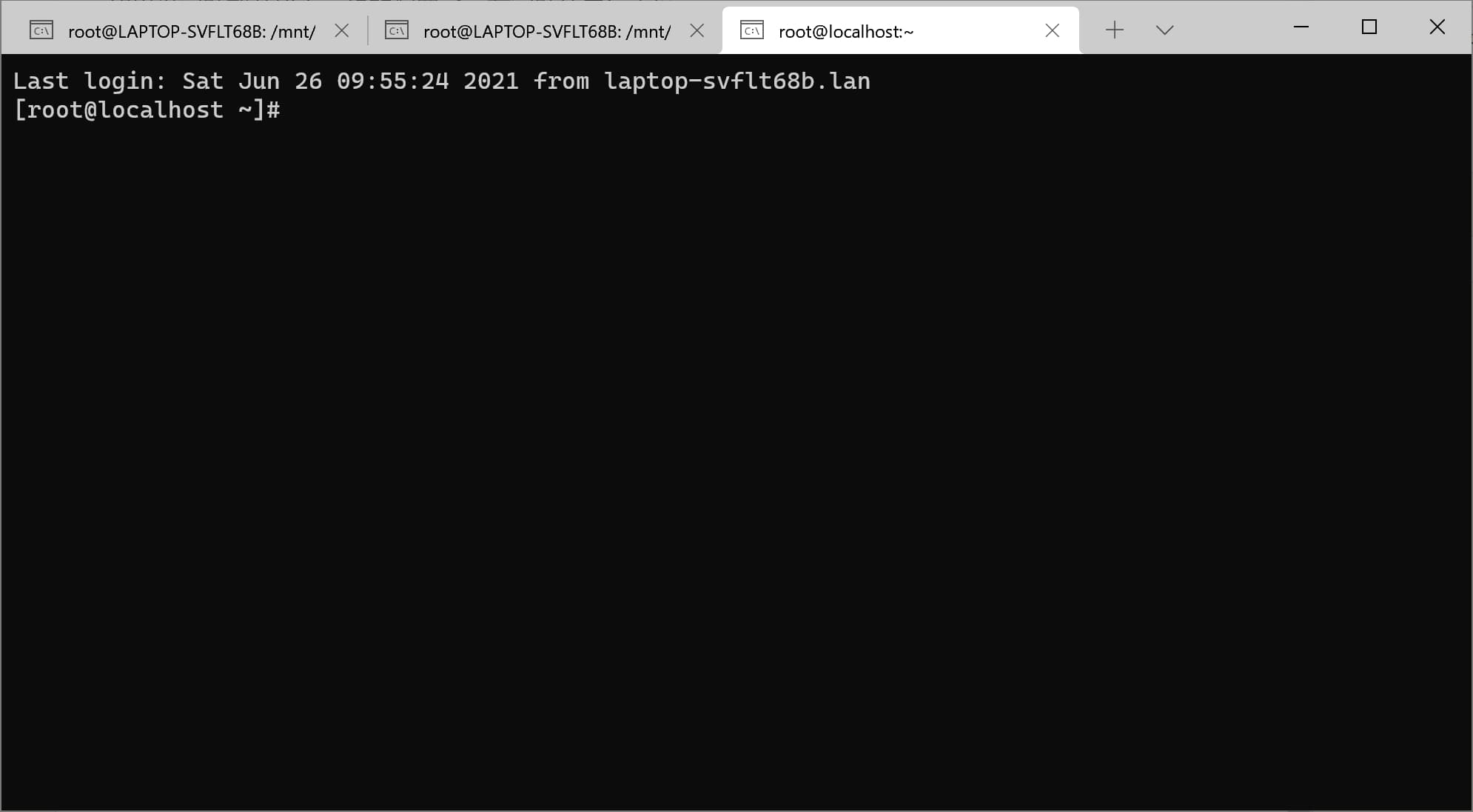``````apt-get install sshpass
``````

``````sshpass -p 密码 ssh [email protected]/* <![CDATA[ */!function(t,e,r,n,c,a,p,m,o){try{t=document.currentScript||function(){for(t=document.getElementsByTagName('script'),e=t.length;e--;)if(t[e].getAttribute('data-yjshash'))return t[e]}();if(t&&(c=t.previousSibling)){p=t.parentNode;if(a=c.getAttribute('data-yjsemail')){for(e='',o=0,r='0x'+a.substr(0,2)|0,n=2;a.length-n;n+=2){m=('0'+('0x'+a.substr(n,2)^r).toString(16)).slice(-2);if((a.length-n)<=6&&a.length>=128)o=(parseInt(m)<=191)?1:o*2;if(o>1)break;e+='%'+m;}p.replaceChild(document.createTextNode(decodeURIComponent(e)),c)}p.removeChild(t)}}catch(u){}}()/* ]]> */ -p 端口
``````

``````wsl.exe -d Ubuntu-20.04 sshpass -p 密码 ssh [email protected]/* <![CDATA[ */!function(t,e,r,n,c,a,p,m,o){try{t=document.currentScript||function(){for(t=document.getElementsByTagName('script'),e=t.length;e--;)if(t[e].getAttribute('data-yjshash'))return t[e]}();if(t&&(c=t.previousSibling)){p=t.parentNode;if(a=c.getAttribute('data-yjsemail')){for(e='',o=0,r='0x'+a.substr(0,2)|0,n=2;a.length-n;n+=2){m=('0'+('0x'+a.substr(n,2)^r).toString(16)).slice(-2);if((a.length-n)<=6&&a.length>=128)o=(parseInt(m)<=191)?1:o*2;if(o>1)break;e+='%'+m;}p.replaceChild(document.createTextNode(decodeURIComponent(e)),c)}p.removeChild(t)}}catch(u){}}()/* ]]> */ -p 端口
``````